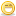1. WATJunior Member

Join Date
Apr 2008
Posts
22

##pipe diameter

I need to calculate pipe diameter for natural gas pipe line as the following details

Flow rate : 37000 m3/h (at 0.00 g)
presure : 7 bar
Gas temperatueer : 20 C
pipe length : 6000 m
pipe type : steel

thanks in avance

See More: pipe diameterReply With Quote

2. Join Date
Always
Posts
Many

3.Senior Member

Join Date
Dec 2007
Posts
114
Thank YouReply With Quote

4. -

5.Member

Join Date
May 2008
Posts
39
You need to use PIPESIM 2003 whose link in available on this forum to find the optimum size...

It depends how much you need pressure at the end!!! and how is the pipeline layed? buried or non-buried?, how is the pipeline elevation profile, how much is the ambient temp,...etc

If you still have problem, get back to meReply With Quote

6.Junior Member

Join Date
Feb 2008
Posts
6
you can use olga 2000 for simulation and you need pvtsim for use the table fluidReply With Quote

7.Junior Member

Join Date
Nov 2008
Posts
24
if you are doing according to ASME then refer ansi B31.2 where you can find formula for pipe thickness calculation....Reply With Quote

8.Junior Member

Join Date
Nov 2008
Posts
24
Any way you can get the outer diameter of the pipe from the vendor or you can take the diameter of the nozzle of the tanK or pressure vessel....Then you can place your tappings or reducers or any other components according to your distribution and pressure you require at the end...Reply With Quote

9.Junior Member

Join Date
Oct 2008
Posts
11
Well firstly, Pipe diameter are depends on Flowrate, Velocity, Compressibility, temperature and Pressure. Here is the equation/formula that i ussualy use for pipe sizing calculation. But the most important thing is that you have to design the velocity. (velocity limitation : below acceptable noise levels, it's about 60 to 80 ft/sec and if the gas contains liquids we have to refer to API RP 14 E which is the minimum velocity should be limited to about 10 ft/sec).

V = 1440 * Z * Q * T / ( d * d * P )
Where V =velocity ft/sec
Z = compressibility (ussualy 0.94)
Q = Flowrate, million cu. ft/hr
T = temperature, R
d = inside diameter of pipe, inch
P = operating pressure, Psia

Good luck ^_^Reply With Quote

10.Junior Member

Join Date
Oct 2008
Posts
11
Well firstly, Pipe diameter are depends on Flowrate, Velocity, Compressibility, temperature and Pressure. Here is the equation/formula that i ussualy use for pipe sizing calculation. But the most important thing is that you have to design the velocity. (velocity limitation : below acceptable noise levels, it's about 60 to 80 ft/sec and if the gas contains liquids we have to refer to API RP 14 E which is the minimum velocity should be limited to about 10 ft/sec).

V = 1440 * Z * Q * T / ( d * d * P )
Where V =velocity ft/sec
Z = compressibility (ussualy 0.94)
Q = Flowrate, million cu. ft/hr
T = temperature, R
d = inside diameter of pipe, inch
P = operating pressure, Psia

Good luck ^_^Reply With Quote

11.Junior Member

Join Date
Jun 2008
Posts
13Originally Posted by WATI need to calculate pipe diameter for natural gas pipe line as the following details

Flow rate : 37000 m3/h (at 0.00 g)
presure : 7 bar
Gas temperatueer : 20 C
pipe length : 6000 m
pipe type : steel

thanks in avance
Usa la eucaci&#243;n de Wymouth que es:qsc=433.9*[tsc/psc]*[[p1^2-p2^2]*d^5.333/[s*L*Tm*Zm]]^0.5=433.9*(536.67/14.7)*((101.55^2-14.7^2)*d^5.333*0.9/(0.9*3.73*527*0.94))^0.5*Eficiencia de tuber&#237;a.
Zm0 factor de compresibilidd 0.94 t&#237;pico, Tm temperatura promedio °R de la tuber&#237;a,tsc temperatura a condiciones estandar °R, psc psia, presi&#243;n a condiciones estandard, L longitud de tuber&#237;a millas,p1 presi&#243;n de entrada, p2 presion de salida de la tuber&#237;a.
d diametro interno de tuber&#237;a in, d= 12.8 in, debes encontrarlos datos correctos, como gravedad espec&#237;fica, t de entrada y salida, presion de entrada y salida, puedes usar Pandhandle, A o B y te dar&#225; el di&#225;metro interior de la tuber&#237;aReply With Quote

12.Junior Member

Join Date
Jun 2008
Posts
13Originally Posted by Guillermo Culebro NievesUsa la eucaci&#243;n de Wymouth que es:qsc=433.9*[tsc/psc]*[[p1^2-p2^2]*d^5.333/[s*L*Tm*Zm]]^0.5=433.9*(536.67/14.7)*((101.55^2-14.7^2)*d^5.333*0.9/(0.9*3.73*527*0.94))^0.5*Eficiencia de tuber&#237;a.
Zm0 factor de compresibilidd 0.94 t&#237;pico, Tm temperatura promedio °R de la tuber&#237;a,tsc temperatura a condiciones estandar °R, psc psia, presi&#243;n a condiciones estandard, L longitud de tuber&#237;a millas,p1 presi&#243;n de entrada, p2 presion de salida de la tuber&#237;a.
d diametro interno de tuber&#237;a in, d= 12.8 in, debes encontrarlos datos correctos, como gravedad espec&#237;fica, t de entrada y salida, presion de entrada y salida, puedes usar Pandhandle, A o B y te dar&#225; el di&#225;metro interior de la tuber&#237;a
qsc
ft^3/dia, ese dato es 31359427(37000m^3/h)Reply With Quote

0.00, 20, 6000, avance, bar, diameter, gas, length, m3/h, natural, pipe, presure, steel, temperatueer, type